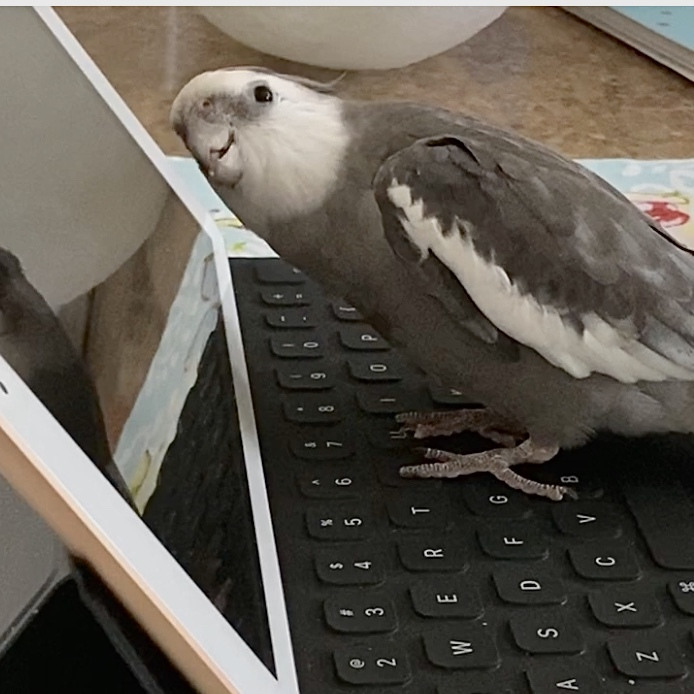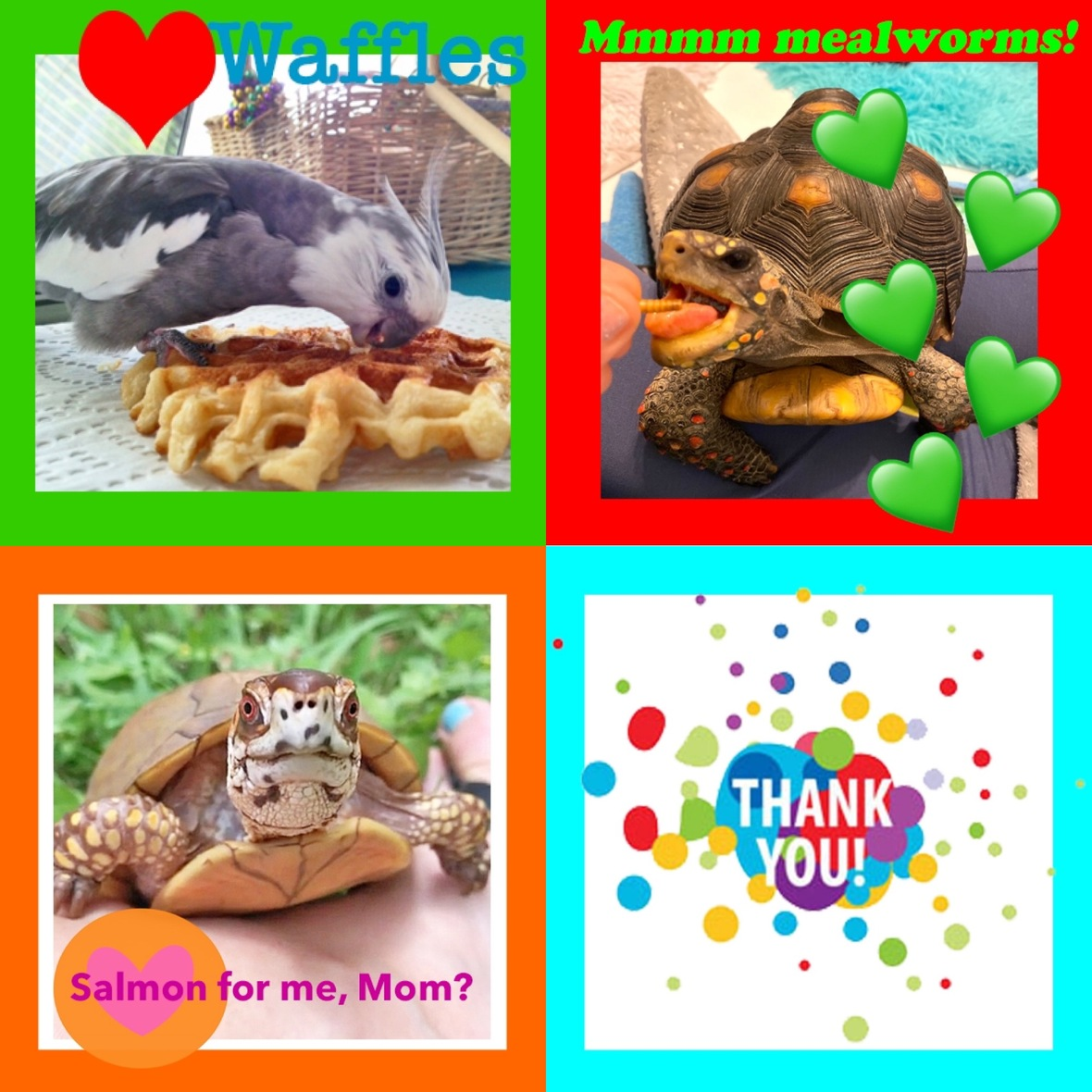Like   Tweet   Pin   +1   in

# Love & Feathers & Shells & Me

## Sept 2020

 table div table+table+table div table{width:100%;padding:0}table div table+table+table div table img{width:96.23%;padding:0;float:none}table div table+table+table div table td{width:100%;padding:0 1.88% 18px}/* styles *//* styles */ Receiving this email as a forward? Sign up to keep following our little flock's adventures!
 table div table+table+table+table+table+table div table{width:100%;padding:0}table div table+table+table+table+table+table div table img{width:96.23%;padding:0;float:none}table div table+table+table+table+table+table div table td{width:100%;padding:0 1.88% 18px}/* styles */table.module-6{width:75.66%;padding:0}table div table+table+table+table+table+table+table div table{width:75.66%;float:none;margin-left:auto;margin-right:auto;padding:0}table div table+table+table+table+table+table+table div table a{border:0 none;text-decoration:none}table div table+table+table+table+table+table+table div table img{width:100%!important;border:0 none;text-decoration:none}table div table+table+table+table+table+table+table div table td{width:100%;padding:0}/* styles */
 table div table+table+table+table+table+table+table+table div table{width:100%;padding:0}table div table+table+table+table+table+table+table+table div table img{width:96.23%;padding:0;float:none}table div table+table+table+table+table+table+table+table div table td{width:100%;padding:0 1.88% 18px}/* styles */table div table+table+table+table+table+table+table+table+table div table,table.module-8{width:37.36%;float:right;padding:0}table div table+table+table+table+table+table+table+table+table div table a{border:0 none;text-decoration:none}table div table+table+table+table+table+table+table+table+table div table img{width:100%!important;border:0 none;text-decoration:none}table div table+table+table+table+table+table+table+table+table div table td{width:100%;padding:0 0 20px 20px}/* styles */ Do you feel brave? I know I do right about now. But it's not because I've conquered all of my big fears or because I am anywhere close to achieving a bucket list goal. It is because I keep waking up and showing up. And that is because my heart is full of love. A wise mentor once said that it takes great strength to withstand struggle. But it takes even greater strength to hold joy. As I watch my precious interspecies flock going about their daily lives so chock-full of trust that all of their daily needs will be met in full, I realize I have been strong enough to hold struggle for many, many moons now. But I am also - at very long last - finally becoming strong enough to hold joy. And speaking of joy and love, even as I type this, a certain feathery flock "alpha" is singing his most alluring song to the newest electronic (and oh so reflective) flock member, his mama's iPad. Who are we kidding. It's his iPad. (And I think the laptop is starting to get a tiny bit jealous.) On a different topic, I am proud to report that a certain red-dotted young lady has managed to destroy yet another fern - this time, one that I literally thought was un-killable. It was called the Macho Fern. It was so enormous that when I first installed it, its fronds covered half her secure outdoor overnight enclosure. Not anymore. She took it down in less than a week. And by down, I mean DOWN. Like...all the way down. Like, imagine a pancake. Now imagine the only ingredient in the pancake batter is a bright green Macho Fern. What can I say. Malti is a pro. On a happier note, while Malti was busy fern-wrangling, Bruce finally got his turn to stretch his (adorable, yellow-dotted) legs in the no-longer-new yet still much-improved outdoor enclosed day play area. Thus far, his favorite activity while he is in there is sitting perfectly still under big piles of bamboo leaf litter. It could be a coincidence, but this also happens to be his favorite activity when he is in his regular enclosure. The one refreshing change is that since there is a whole 10' x 5' area of bamboo leaf litter to burrow into and hide his iPhone-sized, bamboo-colored box turtle body under in the larger day play area, his large shell-less MommyGuard is getting a lot more leg-stretching time while playing "find the box turtle" with him when he takes his turn. Fun, fun, fun. With only a splash of panic. So that's our news. How are you? Are you hanging in there? How is your flock? Our flock sends you mucho mucho cuteness, prayers for even more brave, and love. Always love.

==========

## Flash Gordon, the Tall Tree & the Small Chef

 table div table+table+table+table+table+table+table+table+table+table+table div table{width:100%;padding:0}table div table+table+table+table+table+table+table+table+table+table+table div table img{width:96.23%;padding:0;float:none}table div table+table+table+table+table+table+table+table+table+table+table div table td{width:100%;padding:0 1.88% 18px}/* styles */# Free Tunes for Tough Times

 table div table+table+table+table+table+table+table+table+table+table+table+table+table div table{width:100%;padding:0}table div table+table+table+table+table+table+table+table+table+table+table+table+table div table table{padding:0;float:left!important;width:51.886%!important}table div table+table+table+table+table+table+table+table+table+table+table+table+table div table table+table td,table div table+table+table+table+table+table+table+table+table+table+table+table+table div table td{padding-left:0;padding-right:0}table div table+table+table+table+table+table+table+table+table+table+table+table+table div table table td{padding-left:0;padding-right:20px}table div table+table+table+table+table+table+table+table+table+table+table+table+table div table table+table{float:left!important;width:48.114%!important}/* styles */
 table div table+table+table+table+table+table+table+table+table+table+table+table+table+table div table{width:100%;padding:0}table div table+table+table+table+table+table+table+table+table+table+table+table+table+table div table img{width:96.23%;padding:0;float:none}table div table+table+table+table+table+table+table+table+table+table+table+table+table+table div table td{width:100%;padding:0 1.88% 18px}/* styles */table div table+table+table+table+table+table+table+table+table+table+table+table+table+table+table div table td,table.module-14{width:100%;padding:0}table div table+table+table+table+table+table+table+table+table+table+table+table+table+table+table div table{width:100%;float:none;margin-left:auto;margin-right:auto;padding:0}table div table+table+table+table+table+table+table+table+table+table+table+table+table+table+table div table a{border:0 none;text-decoration:none}table div table+table+table+table+table+table+table+table+table+table+table+table+table+table+table div table img{width:100%!important;border:0 none;text-decoration:none}/* styles */

# Making the laptop jealous.table div table+table+table+table+table+table+table+table+table+table+table+table+table+table+table+table+table+table+table div table{width:100%;padding:0}table div table+table+table+table+table+table+table+table+table+table+table+table+table+table+table+table+table+table+table div table img{width:96.23%;padding:0;float:none}table div table+table+table+table+table+table+table+table+table+table+table+table+table+table+table+table+table+table+table div table td{width:100%;padding:0 1.88% 18px}/* styles */# Macho fern? What Macho fern?

 table.module-20{width:82.08%;padding:0}table div table+table+table+table+table+table+table+table+table+table+table+table+table+table+table+table+table+table+table+table+table div table{width:82.08%;float:none;margin-left:auto;margin-right:auto;padding:0}table div table+table+table+table+table+table+table+table+table+table+table+table+table+table+table+table+table+table+table+table+table div table a{border:0 none;text-decoration:none}table div table+table+table+table+table+table+table+table+table+table+table+table+table+table+table+table+table+table+table+table+table div table img{width:100%!important;border:0 none;text-decoration:none}table div table+table+table+table+table+table+table+table+table+table+table+table+table+table+table+table+table+table+table+table+table div table td{width:100%;padding:0}/* styles */
 table div table+table+table+table+table+table+table+table+table+table+table+table+table+table+table+table+table+table+table+table+table+table+table div table{width:100%;padding:0}table div table+table+table+table+table+table+table+table+table+table+table+table+table+table+table+table+table+table+table+table+table+table+table div table img{width:96.23%;padding:0;float:none}table div table+table+table+table+table+table+table+table+table+table+table+table+table+table+table+table+table+table+table+table+table+table+table div table td{width:100%;padding:0 1.88% 18px}/* styles */# Box turtle hide-n-seek. It's a thing.

 table.module-24{width:75.47%;padding:0}table div table+table+table+table+table+table+table+table+table+table+table+table+table+table+table+table+table+table+table+table+table+table+table+table+table div table{width:75.47%;float:none;margin-left:auto;margin-right:auto;padding:0}table div table+table+table+table+table+table+table+table+table+table+table+table+table+table+table+table+table+table+table+table+table+table+table+table+table div table a{border:0 none;text-decoration:none}table div table+table+table+table+table+table+table+table+table+table+table+table+table+table+table+table+table+table+table+table+table+table+table+table+table div table img{width:100%!important;border:0 none;text-decoration:none}table div table+table+table+table+table+table+table+table+table+table+table+table+table+table+table+table+table+table+table+table+table+table+table+table+table div table td{width:100%;padding:0}/* styles */
 table div table+table+table+table+table+table+table+table+table+table+table+table+table+table+table+table+table+table+table+table+table+table+table+table+table+table+table div table{width:100%;padding:0}table div table+table+table+table+table+table+table+table+table+table+table+table+table+table+table+table+table+table+table+table+table+table+table+table+table+table+table div table img{width:96.23%;padding:0;float:none}table div table+table+table+table+table+table+table+table+table+table+table+table+table+table+table+table+table+table+table+table+table+table+table+table+table+table+table div table td{width:100%;padding:0 1.88% 18px}/* styles */# Feeling the love (& feathers & shells)? Send us a snack!

## Did you know Pearl, Malti & Bruce have a snack fund?

(they're always hungry, too)Click to send a snack to your fav flock member!
 table div table+table+table+table+table+table+table+table+table+table+table+table+table+table+table+table+table+table+table+table+table+table+table+table+table+table+table+table+table+table+table div table{width:100%;padding:0}table div table+table+table+table+table+table+table+table+table+table+table+table+table+table+table+table+table+table+table+table+table+table+table+table+table+table+table+table+table+table+table div table img{width:96.23%;padding:0;float:none}table div table+table+table+table+table+table+table+table+table+table+table+table+table+table+table+table+table+table+table+table+table+table+table+table+table+table+table+table+table+table+table div table td{width:100%;padding:0 1.88% 18px}/* styles */table div table+table+table+table+table+table+table+table+table+table+table+table+table+table+table+table+table+table+table+table+table+table+table+table+table+table+table+table+table+table+table+table div table,table.module-31{width:42.83%;float:right;padding:0}table div table+table+table+table+table+table+table+table+table+table+table+table+table+table+table+table+table+table+table+table+table+table+table+table+table+table+table+table+table+table+table+table div table a{border:0 none;text-decoration:none}table div table+table+table+table+table+table+table+table+table+table+table+table+table+table+table+table+table+table+table+table+table+table+table+table+table+table+table+table+table+table+table+table div table img{width:100%!important;border:0 none;text-decoration:none}table div table+table+table+table+table+table+table+table+table+table+table+table+table+table+table+table+table+table+table+table+table+table+table+table+table+table+table+table+table+table+table+table div table td{width:100%;padding:0 0 20px 20px}/* styles */ Hi! It is so good to meet you here each month! Pearl, Malti, Bruce and their mommy love having you in our extended flock! We are super excited about our newest book, "Waffles & More: A Love & Feathers Recipe Book. This book features 70 of Pearl's favorite "people food" recipes, courtesy of his personal Small Chef (aka his doting large featherless Grandma). Have you treated yourself yet to a copy of "Love & Feathers: what a palm-sized parrot has taught me about life, love, and healthy self-esteem?" If not, it's time! Get ready to smile, laugh, and fall in love with your own pets all over again. ==> To connect with me: www.shannoncutts.com ==> To connect with Pearl, Malti & Bruce: www.loveandfeathersandshells.com
 table div table+table+table+table+table+table+table+table+table+table+table+table+table+table+table+table+table+table+table+table+table+table+table+table+table+table+table+table+table+table+table+table+table div table{width:100%;padding:0}table div table+table+table+table+table+table+table+table+table+table+table+table+table+table+table+table+table+table+table+table+table+table+table+table+table+table+table+table+table+table+table+table+table div table img{width:96.23%;padding:0;float:none}table div table+table+table+table+table+table+table+table+table+table+table+table+table+table+table+table+table+table+table+table+table+table+table+table+table+table+table+table+table+table+table+table+table div table td{width:100%;padding:0 1.88% 18px}/* styles */## Did you know I'm a professional freelance writer?

 /* styles */ If you need fast and fantastic freelance content to support the work you do, or if your company is on the hunt for a copywriter, let's chat! Just hit "reply" to this e-newsletter to let me know you'd like to set up a time to talk. BONUS: Free meet-and-greet with a certain set of enthusiastic feathers is automatically included with every new client Zoom, Skype or call (just try and stop him).
 table.module-35{width:93.21%;padding:0}table div table+table+table+table+table+table+table+table+table+table+table+table+table+table+table+table+table+table+table+table+table+table+table+table+table+table+table+table+table+table+table+table+table+table+table+table div table{width:93.21%;float:none;margin-left:auto;margin-right:auto;padding:0}table div table+table+table+table+table+table+table+table+table+table+table+table+table+table+table+table+table+table+table+table+table+table+table+table+table+table+table+table+table+table+table+table+table+table+table+table div table a{border:0 none;text-decoration:none}table div table+table+table+table+table+table+table+table+table+table+table+table+table+table+table+table+table+table+table+table+table+table+table+table+table+table+table+table+table+table+table+table+table+table+table+table div table img{width:100%!important;border:0 none;text-decoration:none}table div table+table+table+table+table+table+table+table+table+table+table+table+table+table+table+table+table+table+table+table+table+table+table+table+table+table+table+table+table+table+table+table+table+table+table+table div table td{width:100%;padding:0}/* styles */
 table div table+table+table+table+table+table+table+table+table+table+table+table+table+table+table+table+table+table+table+table+table+table+table+table+table+table+table+table+table+table+table+table+table+table+table+table+table div table{width:100%;padding:0}table div table+table+table+table+table+table+table+table+table+table+table+table+table+table+table+table+table+table+table+table+table+table+table+table+table+table+table+table+table+table+table+table+table+table+table+table+table div table img{width:96.23%;padding:0;float:none}table div table+table+table+table+table+table+table+table+table+table+table+table+table+table+table+table+table+table+table+table+table+table+table+table+table+table+table+table+table+table+table+table+table+table+table+table+table div table td{width:100%;padding:0 1.88% 18px}/* styles */table.module-37{width:99.81%;padding:0}table div table+table+table+table+table+table+table+table+table+table+table+table+table+table+table+table+table+table+table+table+table+table+table+table+table+table+table+table+table+table+table+table+table+table+table+table+table+table div table{width:99.81%;float:none;margin-left:auto;margin-right:auto;padding:0}table div table+table+table+table+table+table+table+table+table+table+table+table+table+table+table+table+table+table+table+table+table+table+table+table+table+table+table+table+table+table+table+table+table+table+table+table+table+table div table a{border:0 none;text-decoration:none}table div table+table+table+table+table+table+table+table+table+table+table+table+table+table+table+table+table+table+table+table+table+table+table+table+table+table+table+table+table+table+table+table+table+table+table+table+table+table div table img{width:100%!important;border:0 none;text-decoration:none}table div table+table+table+table+table+table+table+table+table+table+table+table+table+table+table+table+table+table+table+table+table+table+table+table+table+table+table+table+table+table+table+table+table+table+table+table+table+table div table td{width:100%;padding:0}/* styles */
 table div table+table+table+table+table+table+table+table+table+table+table+table+table+table+table+table+table+table+table+table+table+table+table+table+table+table+table+table+table+table+table+table+table+table+table+table+table+table+table div table{width:100%;padding:0}table div table+table+table+table+table+table+table+table+table+table+table+table+table+table+table+table+table+table+table+table+table+table+table+table+table+table+table+table+table+table+table+table+table+table+table+table+table+table+table div table img{width:96.23%;padding:0;float:none}table div table+table+table+table+table+table+table+table+table+table+table+table+table+table+table+table+table+table+table+table+table+table+table+table+table+table+table+table+table+table+table+table+table+table+table+table+table+table+table div table td{width:100%;padding:0 1.88% 18px}/* styles */